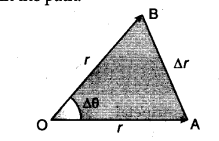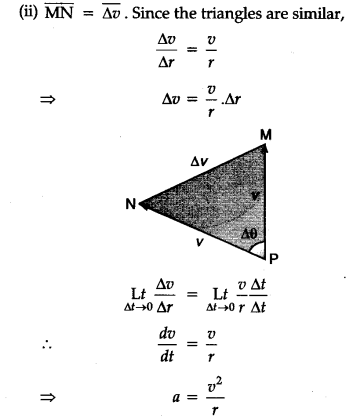# An object is moving on a circular path in horizontal plane. Radius of the paths is r and constant speed is v. Deduce expression for centripetal acceleration

Consider a body in a circular path of radius r, with a speed v. The velocity direction is tangential at any point in the path.The position vectors at A and B are represented by two sides of an isosceles triangle.
(i) The change in position vector is indicated by AB = $\delta$r. The velocity at A and B are along the tangents . at these points and the change in velocity will complete an isosceles triangle of velocities.